# ASRF model

## Definition

ASFR Model (asymptotic single factor risk model) is a simplified Credit Portfolio risk model that underpins the Basel II capital requirements

## Assumptions

The following is an indicative list of assumptions / design choices in constructing the ASFR model

• One year risk horizon
• Single factor model
• Normal distributions
• Homogeneous and large pool of exposures

## Formulae

Below are the explicit formulae for some banks’ major products:

• Corporate exposures,
• Small-medium enterprise (SME) exposures
• Residential mortgages and
• Qualifying revolving retail exposure. (S being Min(Max(Sales Turnover),5),50 )

In the formulas below:

### Corporate Exposures

The exposure for corporate loans is calculated as follows

#### Correlation Parameter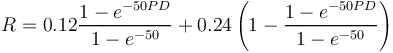$R = 0.12 \frac{1 - e^{-50 PD}}{1 - e^{-50}} + 0.24 \left(1- \frac{1 - e^{-50 PD}}{1 - e^{-50}}\right)$

The correlation parameter offers a simple approach to capture an element of Default Correlation. The peculiar presence of exponentials is for normalization purposes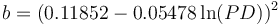$b= (0.11852 - 0.05478 \ln(PD))^2$

The maturity adjustment offers a simple approach to capture residual credit risks that may not materialise within the one-year risk horizon implied by the ASRF model.

#### Capital requirement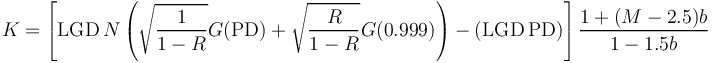$K= \left[\mbox{LGD} \, N\left(\sqrt{\frac{1}{1-R}} G(\mbox{PD}) +\sqrt{\frac{R}{1-R}} G(0.999)\right) - (\mbox{LGD} \, \mbox{PD})\right] \frac{1+(M-2.5) b}{1-1.5 b}$

#### Risk-weighted assets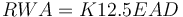$RWA = K 12.5 EAD$

### Correlation adjustment for Large Financial Institutions

A multiplier of 1.25 is applied to the corporate correlation parameter of all exposures to financial institutions meeting the following criteria

• Regulated financial institutions whose total assets are greater than or equal to US \$100 billion. Regulated financial institutions include, but are not limited to, prudentially regulated Insurance Companies, Broker/Dealers, Banks, Thrifts and Futures Commission Merchants
• Unregulated financial institutions, regardless of size.

#### Correlation Parameter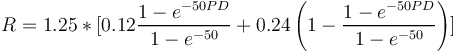$R = 1.25 * [0.12 \frac{1 - e^{-50 PD}}{1 - e^{-50}} + 0.24 \left(1- \frac{1 - e^{-50 PD}}{1 - e^{-50}}\right) ]$

For small and medium enterprises with annual Sales Turnover below 50 million euro, the correlation may be adjusted as follows:

#### SME Correlation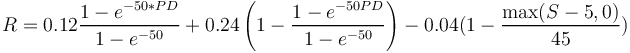$R = 0.12 \frac{1 - e^{-50 * PD}}{1 - e^{-50}} + 0.24 \left(1- \frac{1 - e^{-50 PD}}{1 - e^{-50}}\right) - 0.04 (1-\frac{\max(S-5,0)}{45})$

In the above formula, S is the enterprise's annual sales turnover in millions of euro.

### Residential mortgage exposures

The exposure related to residential mortgages can be calculated as this

#### Correlation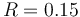$R = 0.15$

#### Capital Requirement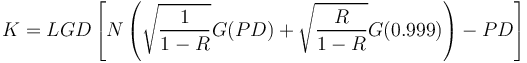$K=LGD \left[N\left(\sqrt{\frac{1}{1-R}} G(PD) +\sqrt{\frac{R}{1-R}} G(0.999)\right) - PD\right]$

#### Risk-weighted assets$RWA = K 12.5 EAD$

### Qualifying revolving retail exposures (credit card products)

The exposure related to unsecured retail credit products can be calculated as follows:

#### QRRE Correlation Assumption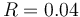$R = 0.04$

#### Capital Requirement$K=LGD \left[N\left(\sqrt{\frac{1}{1-R}} G(PD) +\sqrt{\frac{R}{1-R}} G(0.999)\right) - PD\right]$

#### Risk-weighted assets$RWA = K 12.5 EAD$